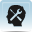# Resource 5: Interesting facts about the earthBackground information / subject knowledge for teacher

This resource provides you with some information about the Earth and about Africa. Get your students to guess the answers. You can also get them to estimate things like each other’s height and weight, the length of the room etc. Being able to estimate measurements is a useful skill in science as it enables scientists to spot errors.

If some of the students in your class are finding this topic easy, you could ask them to calculate the density of the Earth and the Moon.

Density = mass/volume

Volume = 4/3 π r3 (π = 3. 142) r = radius

## The Earth

Diameter = 12,760 km

Mass = 5.972 x 1024 kg

Crust = 40 km thick

Distance from the Earth to the Sun = 1. 426 x 109 km

Distance from Earth to the Moon = 384,000 km

## The Moon

Diameter = 3,475 km